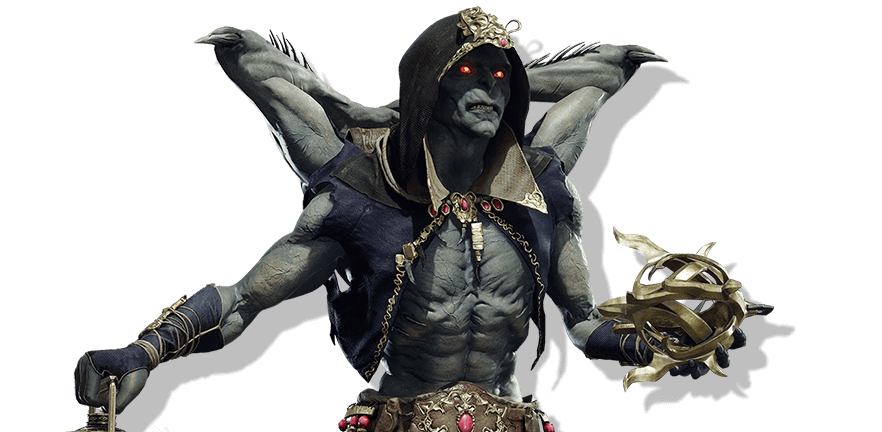Start learning movesets and combos!

Choose the platform you play on.
See how to read and perform inputs.Mortal Kombat 11

# KollectorCombos

The only Naknada representation within the Mortal Kombat roster, Kollector is a loyal subject of Shao Khan.Know more

Get notified of the character’s updates

## Basic combo attacks

Combinations of basic moves

One
Three

One
Three
One

Back
+
One
Two

Back
+
One
Two
Two

Forward
+
One
Two

Forward
+
One
Two
Down
+
One

Forward
+
One
Two
Down
+
Two

Two
One
+
Three

Two
One
+
Three
Four

Forward
+
Two
Two

Forward
+
Two
Two
One
+
Three

Back
+
Two
Three

Back
+
Two
Three
Three

Three
Two

Forward
+
Three
One

Forward
+
Three
One
Two

Forward
+
Three
One
Two
Three

Four
Four

### Ravages Of Time

Four
Four
Three

Long combinations of various moves including abilities and specials

Up
+
Two
Forward
Forward
Forward
+
Two
Two
Down
Back
Forward
Three

Three
Two
KB
Forward
Forward
Forward
+
Two
Two
Down
Back
Forward
Three

Down
Back
Forward
Three
KB
Forward
+
Two
Two
One
+
Three

Up
+
Two
Forward
Forward
Forward
+
Two
Two
One
+
Three

Down
+
Two
KB
Forward
Forward
Down
+
One
Forward
Forward
Forward
+
Two
Two
Down
Back
Three

Three
Two
KB
Forward
Forward
Forward
+
Two
Two
Down
Back
Three

Up
+
Two
Forward
Forward
Forward
+
Two
Two
Down
Back
Three

Down
+
Two
KB
Forward
Forward
Down
+
One
Forward
Forward
Forward
+
Two
Two
Down
Back
Three

Three
Two
KB
Forward
Forward
Forward
+
Two
Two
Down
Back
Three